# Regular dodecahedron

Regular Dodecahedron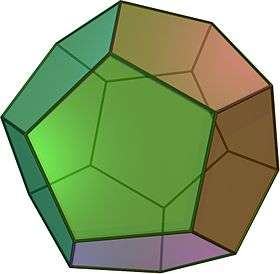TypePlatonic solid
ElementsF = 12, E = 30
V = 20 (χ = 2)
Faces by sides12{5}
Conway notationD
Schläfli symbols{5,3}
Wythoff symbol3 | 2 5
Coxeter diagramSymmetryIh, H3, [5,3], (*532)
Rotation groupI, [5,3]+, (532)
ReferencesU23, C26, W5
PropertiesRegular convex
Dihedral angle116.56505° = arccos(−1/5)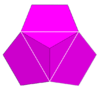5.5.5
(Vertex figure)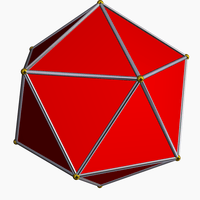Regular icosahedron
(dual polyhedron)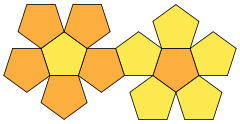Net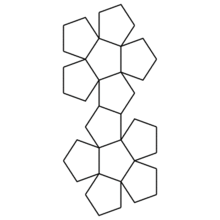Animation of a net of a regular (pentagonal) dodecahedron being folded

A regular dodecahedron or pentagonal dodecahedron is a dodecahedron that is regular composed of twelve regular pentagonal faces, with three meeting at each vertex, and is represented by the Schläfli symbol {5,3}. It is one of the five Platonic solids. It has 20 vertices, 30 edges and 160 diagonals (60 face diagonals, 100 space diagonals).

## Dimensions

If the edge length of a regular dodecahedron is a, the radius of a circumscribed sphere (one that touches the regular dodecahedron at all vertices) isand the radius of an inscribed sphere (tangent to each of the regular dodecahedron's faces) iswhile the midradius, which touches the middle of each edge, isThese quantities may also be expressed aswhere ϕ is the golden ratio.

Note that, given a regular dodecahedron of edge length one, ru is the radius of a circumscribing sphere about a cube of edge length ϕ, and ri is the apothem of a regular pentagon of edge length ϕ.

## Area and volume

The surface area A and the volume V of a regular dodecahedron of edge length a are:## Two-dimensional symmetry projections

The regular dodecahedron has two special orthogonal projections, centered, on vertices and pentagonal faces, correspond to the A2 and H2 Coxeter planes.

Orthogonal projections
Centered by Vertex Edge Face
Image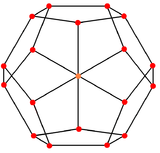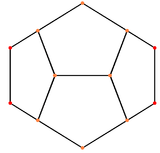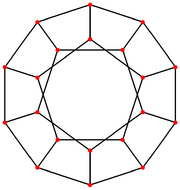Projective
symmetry
[] =   [] = 

In perspective projection, viewed above a pentagonal face, the regular dodecahedron can be seen as a linear-edged schlegel diagram, or stereographic projection as a spherical polyhedron. These projections are also used in showing the four-dimensional 120-cell, a regular 4-dimensional polytope, constructed from 120 dodecahedra, projecting it down to 3-dimensions.

Projection Orthogonal projection Perspective projection
Schlegel diagram Stereographic projection
Regular dodecahedron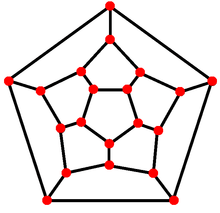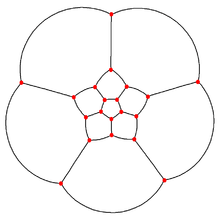Dodecaplex
(120-cell)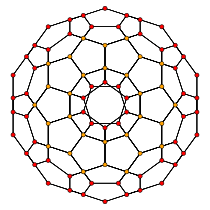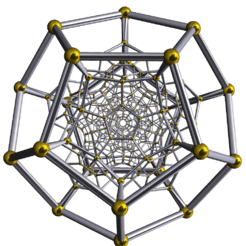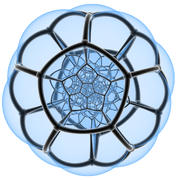## Spherical tiling

The regular dodecahedron can also be represented as a spherical tiling.

Orthographic projection Stereographic projection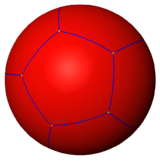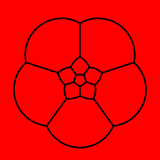## Cartesian coordinates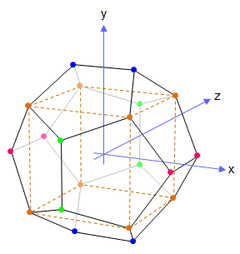Vertex coordinates: The orange vertices lie at (±1, ±1, ±1) and form a cube (dotted lines). The green vertices lie at (0, ±1/ϕ, ±ϕ) and form a rectangle on the yz-plane. The blue vertices lie at (±1/ϕ, ±ϕ, 0) and form a rectangle on the xy-plane. The pink vertices lie at (±ϕ, 0, ±1/ϕ) and form a rectangle on the xz-plane. The distance between adjacent vertices is 2/ϕ, and the distance from the origin to any vertex is √3.ϕ = 1 + √5/2 is the golden ratio.

The following Cartesian coordinates define the 20 vertices of a regular dodecahedron centered at the origin and suitably scaled and oriented:

(±1, ±1, ±1)
(0, ±1/ϕ, ±ϕ)
1/ϕ, ±ϕ, 0)
ϕ, 0, ±1/ϕ)

where ϕ = 1 + 5/2 is the golden ratio (also written τ) ≈ 1.618. The edge length is 2/ϕ = 5 − 1. The containing sphere has a radius of 3.

### Facet-defining equations

Similar to the symmetry of the vertex coordinates, the equations of the twelve facets of the regular dodecahedron also display symmetry in their coefficients:

ϕx ± y = ±ϕ2
ϕy ± z = ±ϕ2
ϕz ± x = ±ϕ2

## Properties

• The dihedral angle of a regular dodecahedron is 2 arctan(ϕ) or approximately 116.5650512° (where again ϕ = 1 + 5/2, the golden ratio).
• If the original regular dodecahedron has edge length 1, its dual icosahedron has edge length ϕ.
• If the five Platonic solids are built with same volume, the regular dodecahedron has the shortest edges.
• It has 43,380 nets.
• The map-coloring number of a regular dodecahedron's faces is 4.
• The distance between the vertices on the same face not connected by an edge is ϕ times the edge length.
• If two edges share a common vertex, then the midpoints of those edges form an equilateral triangle with the body center.

## Geometric relations

The regular dodecahedron is the third in an infinite set of truncated trapezohedra which can be constructed by truncating the two axial vertices of a pentagonal trapezohedron.

The stellations of the regular dodecahedron make up three of the four Kepler–Poinsot polyhedra.

A rectified regular dodecahedron forms an icosidodecahedron.

The regular dodecahedron has icosahedral symmetry Ih, Coxeter group [5,3], order 120, with an abstract group structure of A5 × Z2.

### Relation to the regular icosahedron

When a regular dodecahedron is inscribed in a sphere, it occupies more of the sphere's volume (66.49%) than an icosahedron inscribed in the same sphere (60.54%).

A regular dodecahedron with edge length 1 has more than three and a half times the volume of an icosahedron with the same length edges (7.663... compared with 2.181...), which ratio is approximately 3.51246117975, or in exact terms: 3/5(3ϕ + 1) or (1.8ϕ + 0.6).

A regular dodecahedron has 12 faces and 20 vertices, whereas a regular icosahedron has 20 faces and 12 vertices. Both have 30 edges.

### Relation to the nested cube

A cube can be embedded within a regular dodecahedron, affixed to eight of its equidistant vertices, in five different positions. In fact, five cubes may overlap and interlock inside the regular dodecahedron to result in the compound of five cubes.

The ratio of the edge of a regular dodecahedron to the edge of a cube embedded inside such a regular dodecahedron is 1 : ϕ, or (ϕ  1) : 1.

The ratio of a regular dodecahedron's volume to the volume of a cube embedded inside such a regular dodecahedron is 1 : 2/2 + ϕ, or 1 + ϕ/2 : 1, or (5 + 5) : 4.

For example, an embedded cube with a volume of 64 (and edge length of 4), will nest within a regular dodecahedron of volume 64 + 32ϕ (and edge length of 4ϕ  4).

Thus, the difference in volume between the encompassing regular dodecahedron and the enclosed cube is always one half the volume of the cube times ϕ.

From these ratios are derived simple formulas for the volume of a regular dodecahedron with edge length a in terms of the golden mean:

V = ()3 · 1/4(5 + 5)
V = 1/4(14ϕ + 8)a3

### Relation to the golden rectangle

Golden ratio rectangles of ratio (ϕ + 1) : 1 and ϕ : 1 also fit perfectly within a regular dodecahedron. In proportion to this golden rectangle, an enclosed cube's edge is ϕ, when the long length of the rectangle is ϕ + 1 (or ϕ2) and the short length is 1 (the edge shared with the regular dodecahedron).

In addition, the center of each face of the regular dodecahedron form three intersecting golden rectangles.

## History and uses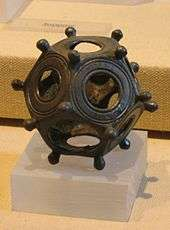Roman dodecahedron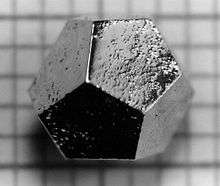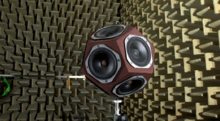Omnidirectional sound source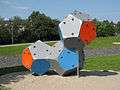A climbing wall consisting of three dodecahedral pieces

Regular dodecahedral objects have found some practical applications, and have also played a role in the visual arts and in philosophy.

Iamblichus states that Hippasus, a Pythagorean, perished in the sea, because he boasted that he first divulged "the sphere with the twelve pentagons." In Theaetetus, a dialogue of Plato, Plato was able to prove that there are just five uniform regular solids; they later became known as the platonic solids. Timaeus (c. 360 B.C.), as a personage of Plato's dialogue, associates the other four platonic solids with the four classical elements, adding that there is a fifth solid pattern which, though commonly associated with the regular dodecahedron, is never directly mentioned as such; "this God used in the delineation of the universe." Aristotle also postulated that the heavens were made of a fifth element, which he called aithêr (aether in Latin, ether in American English).

Regular dodecahedra have been used as dice and probably also as divinatory devices. During the hellenistic era, small, hollow bronze Roman dodecahedra were made and have been found in various Roman ruins in Europe. Their purpose is not certain.

In 20th-century art, dodecahedra appear in the work of M. C. Escher, such as his lithographs Reptiles (1943) and Gravitation (1952). In Salvador Dalí's painting The Sacrament of the Last Supper (1955), the room is a hollow regular dodecahedron. Gerard Caris based his entire artistic oeuvre on the regular dodecahedron and the pentagon, which is presented as a new art movement coined as Pentagonism.

In modern role-playing games, the regular dodecahedron is often used as a twelve-sided die, one of the more common polyhedral dice.

Some quasicrystals have dodecahedral shape (see figure). Some regular crystals such as garnet and diamond are also said to exhibit "dodecahedral" habit, but this statement actually refers to the rhombic dodecahedron shape.

Immersive Media, a camera manufacturing company, has made the Dodeca 2360 camera, the world's first 360°, full motion camera which captures high-resolution video from every direction simultaneously at more than 100 million pixels per second or 30 frames per second. It is based on regular dodecahedron.

The popular puzzle game Megaminx is in the shape of a regular dodecahedron.

In the children's novel The Phantom Tollbooth, the regular dodecahedron appears as a character in the land of Mathematics. Each of his faces wears a different expression—e.g. happy, angry, sad—which he swivels to the front as required to match his mood.

Dodecahedron is the name of an avant-garde black metal band from Netherlands.

### Shape of the universe

Various models have been proposed for the global geometry of the universe. In addition to the primitive geometries, these proposals include the Poincaré dodecahedral space, a positively curved space consisting of a regular dodecahedron whose opposite faces correspond (with a small twist). This was proposed by Jean-Pierre Luminet and colleagues in 2003, and an optimal orientation on the sky for the model was estimated in 2008.

In Bertrand Russell's 1954 short story "The Mathematician's Nightmare: The Vision of Professor Squarepunt," the number 5 said: "I am the number of fingers on a hand. I make pentagons and pentagrams. And but for me dodecahedra could not exist; and, as everyone knows, the universe is a dodecahedron. So, but for me, there could be no universe."

## Space filling with cube and bilunabirotunda

Regular dodecahedra fill space with cubes and bilunabirotundae (Johnson solid 91), in the ratio of 1 to 1 to 3. The dodecahedra alone make a lattice of edge-to-edge pyritohedra. The bilunabirotundae fill the rhombic gaps. Each cube meets six bilunabirotundae in three orientations.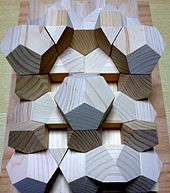Block model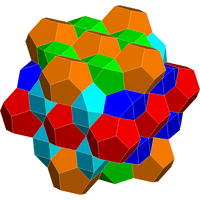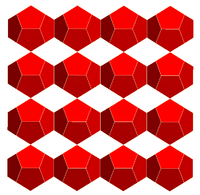Lattice of dodecahedra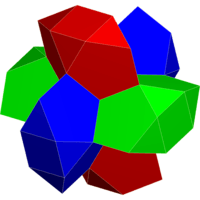6 bilunabirotundae around a cube

The regular dodecahedron is topologically related to a series of tilings by vertex figure n3.

The regular dodecahedron can be transformed by a truncation sequence into its dual, the icosahedron:

The regular dodecahedron is a member of a sequence of otherwise non-uniform polyhedra and tilings, composed of pentagons with face configurations (V3.3.3.3.n). (For n > 6, the sequence consists of tilings of the hyperbolic plane.) These face-transitive figures have (n32) rotational symmetry.

## Vertex arrangement

The regular dodecahedron shares its vertex arrangement with four nonconvex uniform polyhedra and three uniform polyhedron compounds.

Five cubes fit within, with their edges as diagonals of the regular dodecahedron's faces, and together these make up the regular polyhedral compound of five cubes. Since two tetrahedra can fit on alternate cube vertices, five and ten tetrahedra can also fit in a regular dodecahedron.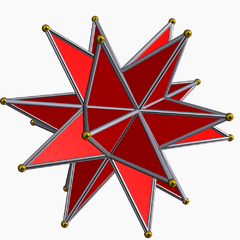Great stellated dodecahedron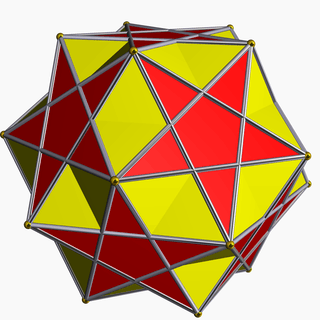Small ditrigonal icosidodecahedron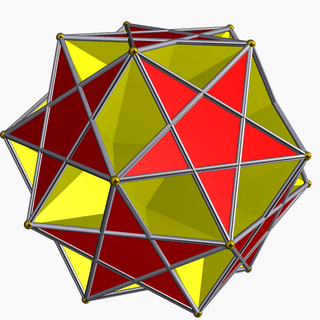Ditrigonal dodecadodecahedron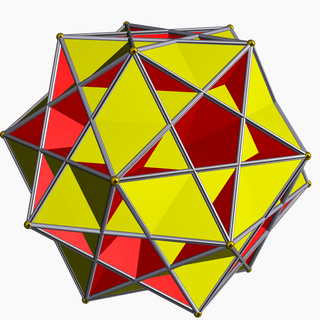Great ditrigonal icosidodecahedron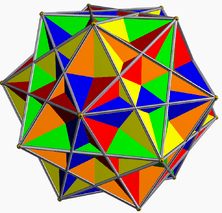Compound of five cubes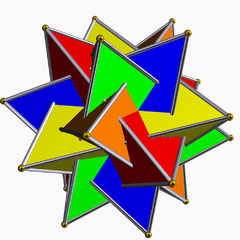Compound of five tetrahedra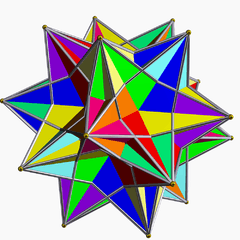Compound of ten tetrahedra

## Stellations

The 3 stellations of the regular dodecahedron are all regular (nonconvex) polyhedra: (Kepler–Poinsot polyhedra)

0 1 2 3
Stellation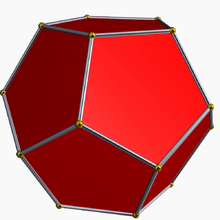Regular dodecahedron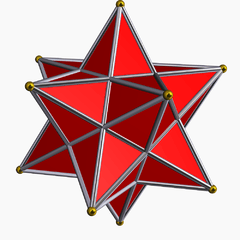Small stellated dodecahedron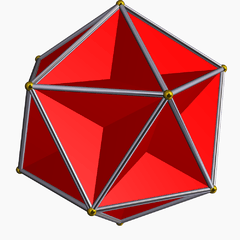Great dodecahedronGreat stellated dodecahedron
Facet diagram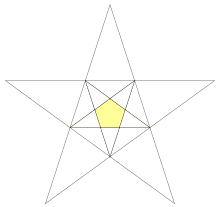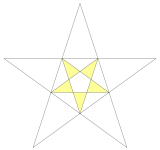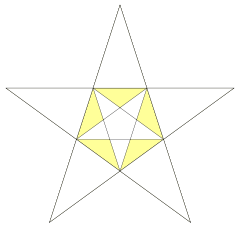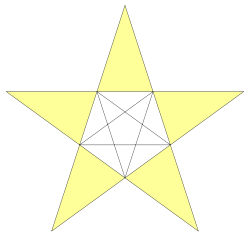## Dodecahedral graph

Regular dodecahedron graph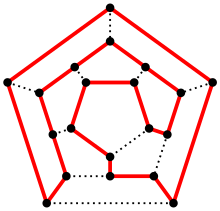A Hamiltonian cycle in a dodecahedron.
Vertices 20
Edges 30
Diameter 5
Girth 5
Automorphisms 120 (S5)
Chromatic number 3
Properties Hamiltonian, regular, symmetric, distance-regular, distance-transitive, 3-vertex-connected, planar graph

The skeleton of the dodecahedron (the vertices and edges) form a graph. It is one of 5 Platonic graphs, each a skeleton of its Platonic solid.

This graph can also be constructed as the generalized Petersen graph G(10,2). The high degree of symmetry of the polygon is replicated in the properties of this graph, which is distance-transitive, distance-regular, and symmetric. The automorphism group has order 120. The vertices can be colored with 3 colors, as can the edges, and the diameter is 5.

The dodecahedral graph is Hamiltonian—there is a cycle containing all the vertices. Indeed, this name derives from a mathematical game invented in 1857 by William Rowan Hamilton, the icosian game. The game's object was to find a Hamiltonian cycle along the edges of a dodecahedron.1. Sutton, Daud (2002), Platonic & Archimedean Solids, Wooden Books, Bloomsbury Publishing USA, p. 55, ISBN 9780802713865.
2. http://mathworld.wolfram.com/images/eps-gif/DodecahedronCube_700.gif
3. http://davidf.faricy.net/polyhedra/images/dodecarect.gif
4. http://www.toshen.com/images/dodecahedronwithgoldrectang.gif
5. Plato, Timaeus, Jowett translation [line 1317–8]; the Greek word translated as delineation is diazographein, painting in semblance of life.
6. Dodecahedral Crystal Habit
7. Dumé, Belle (Oct 8, 2003). "Is The Universe A Dodecahedron?". PhysicsWorld. Archived from the original on 2012-04-25.
8. Luminet, Jean-Pierre; Jeff Weeks; Alain Riazuelo; Roland Lehoucq; Jean-Phillipe Uzan (2003-10-09). "Dodecahedral space topology as an explanation for weak wide-angle temperature correlations in the cosmic microwave background". Nature. 425 (6958): 593–5. arXiv:astro-ph/0310253. Bibcode:2003Natur.425..593L. doi:10.1038/nature01944. PMID 14534579.
9. Roukema, Boudewijn; Zbigniew Buliński; Agnieszka Szaniewska; Nicolas E. Gaudin (2008). "A test of the Poincaré dodecahedral space topology hypothesis with the WMAP CMB data". Astronomy and Astrophysics. 482 (3): 747. arXiv:0801.0006. Bibcode:2008A&A...482..747L. doi:10.1051/0004-6361:20078777.
10. http://demonstrations.wolfram.com/DodecahedronAndBilunabirotunda/
11. http://www.lcv.ne.jp/~hhase/memo/m09_08b.htmlWikimedia Commons has media related to Regular dodecahedra.

Fundamental convex regular and uniform polytopes in dimensions 2–10
Family An Bn I2(p) / Dn E6 / E7 / E8 / E9 / E10 / F4 / G2 Hn
Regular polygon Triangle Square p-gon Hexagon Pentagon
Uniform polyhedron Tetrahedron OctahedronCube Demicube DodecahedronIcosahedron
Uniform 4-polytope 5-cell 16-cellTesseract Demitesseract 24-cell 120-cell600-cell
Uniform 5-polytope 5-simplex 5-orthoplex5-cube 5-demicube
Uniform 6-polytope 6-simplex 6-orthoplex6-cube 6-demicube 122221
Uniform 7-polytope 7-simplex 7-orthoplex7-cube 7-demicube 132231321
Uniform 8-polytope 8-simplex 8-orthoplex8-cube 8-demicube 142241421
Uniform 9-polytope 9-simplex 9-orthoplex9-cube 9-demicube
Uniform 10-polytope 10-simplex 10-orthoplex10-cube 10-demicube
Uniform n-polytope n-simplex n-orthoplexn-cube n-demicube 1k22k1k21 n-pentagonal polytope
Topics: Polytope familiesRegular polytopeList of regular polytopes and compounds